Combining Like Terms

 Problem Set 1 Problem Set 2 Problem Set 3Select a problem set using the buttons above, then use your mouse or tab key to select a question. Fill in the blank with the correct answer. Express your answers with any variable term first. Example: 2 - 3x should be expressed as -3x + 2 When you have answered all of the questions, ask Charlie how you did.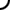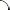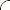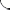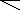Simplify each expression.   2x + 3x 5a - 2a 4y - 2y - 5y 3 + 2 + 7t - 4t 4 - 3m - 1 + 2m 15r - 10 - (-7r) + 5r + 3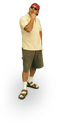Simplify each expression.   -6x + 4x 13a - 20a -8y + 5y - 2y 12t - 9 - t - 4 -5m + 11 - 2m - 4 6 - 5r + 14 + 10r - (-5)Simplify each expression.   -5x + 7x -6a - 9a -4y + 3y - 9y 16 - (-4) + 7t - 11t -15 + m - 14m - 12 -2r + (-3r) + 10 - (-7) + 5r©  2009  LarryPerezWeb Design:  Patrick Quigley